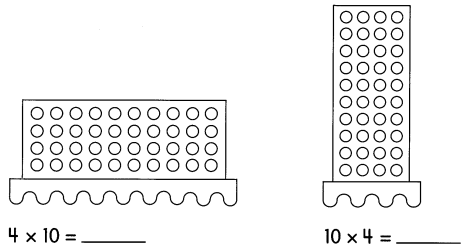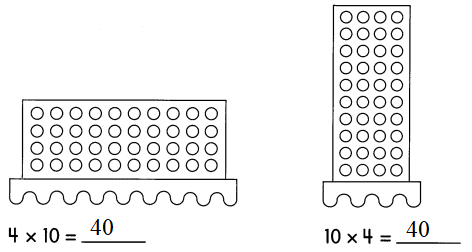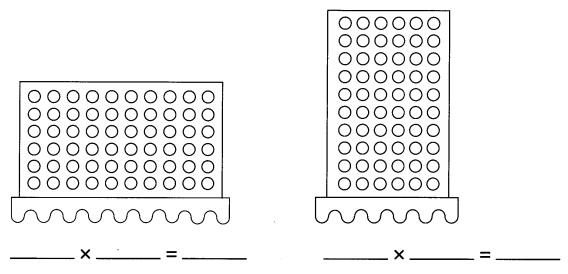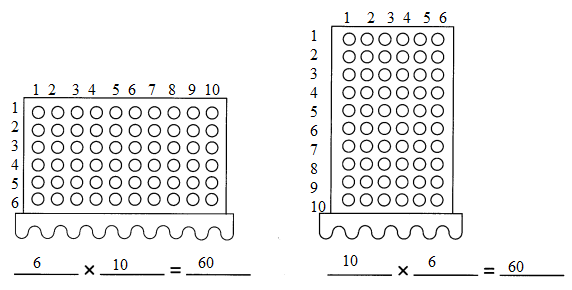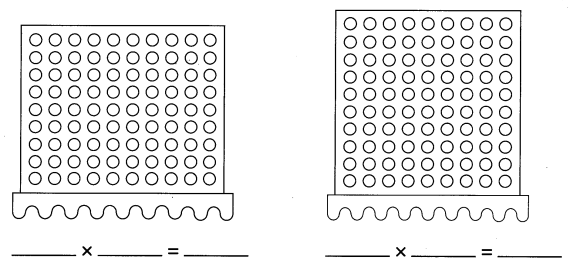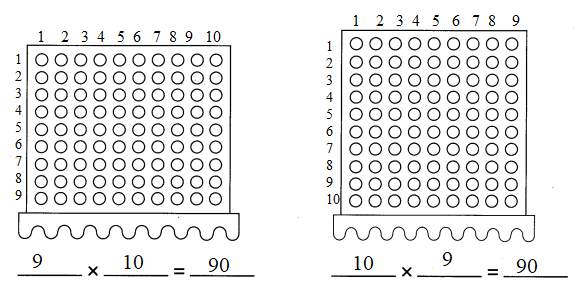# Math in Focus Grade 2 Chapter 6 Practice 5 Answer Key Multiplying 10: Skip-counting and Using Dot Paper

Go through the Math in Focus Grade 2 Workbook Answer Key Chapter 6 Practice 5 Multiplying 10: Skip-counting and Using Dot Paper to finish your assignments.

## Math in Focus Grade 2 Chapter 6 Practice 5 Answer Key Multiplying 10: Skip-counting and Using Dot Paper

The numbers below the chart follow a pattern. Use the hundreds chart to find the missing numbers.

Use patterns to fill in the blanks.

Question 1.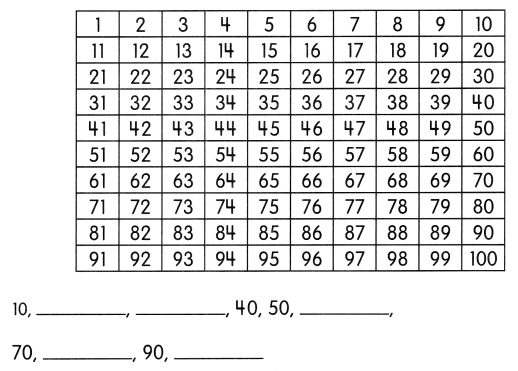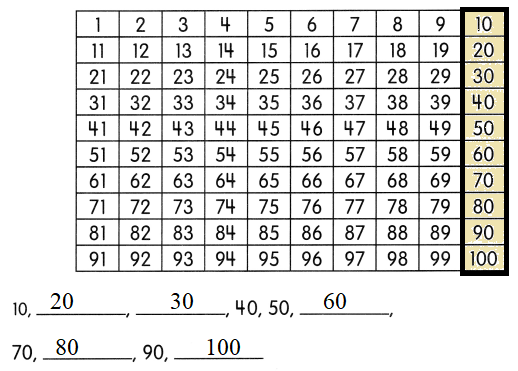Question 2.
2 × 1 = ___
2 × 10 = ____
2 × 1 = 2
2 × 10 = 20

Question 3.
3 × 1 = ___
3 × 10 = ___
3 × 1 = 3
3 × 10 = 30

Use patterns to fill in the blanks.

Question 4.
4 × 1 = ____
4 × 10 = ___
4 × 1 = 4
4 × 10 = 40

Question 5.
5 × 1 = _____
5 × 10 = ___
5 × 1 = 5
5 × 10 = 50

Question 6.
6 × 10 = ___
6 × 10 = 60

Question 7.
7 × 10 = ___
7 × 10 = 70

Solve.

Question 8.
There are 4 bundles of sticks. Each bundle has 10 sticks. How many sticks are there in all?

4 × 10 = ____
There are ___ sticks in all.
4 × 10 = 40
There are 40 sticks in all

Question 9.
Megan makes 6 bracelets. She needs 10 beads to make one bracelet. How many beads are needed to make the 6 bracelets?
___ × 10 = ___
____ beads are needed to make the 6 bracelets.
6 × 10 = 60
60 beads are needed to make the 6 bracelets.

Solve.

Question 10.
During sports day, 10 children form a group for a relay race. How many children are there in 8 groups?
____ × 10 = _____
There are ___ children in 8 groups.
8 × 10 = 80
There are 20 children in 8 groups.

Question 11.
The school band has 10 violins. Each violin has 4 strings on it. How many strings are on the 10 violins?
____ × ___ = ____
There are ___ strings on the 10 violins.
1 0 × 4 = 40
There are 40 strings on the 10 violins.

Use dot paper to multiply.

Question 12.